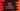# Python program to check if a subarray is in an array## Python program to check if a subarray is in an array:

This post will show you how to check if a subarray is in another array using python. For example, if the first array is {2, 4, 5, 7, 8, 0} and if the second array is {5, 7, 8}, it will print true.

### Algorithm:

The best way to solve this is by using two pointer variables. Both pointers will first points to the first element of the arrays. The pointer of the second array will move to next only if the first element of the second array is equal to the first element of the first array. Once matches, we will increment both pointers of both arrays simultaneously. If any value doesn’t match, we will reset the second array pointer to 0.

If all the elements of the second array matches with the elements of the first array, the check is passed.

### Python program:

Below is the complete python program:

``````def find_subarray(first_arr, second_arr):
first_ptr = 0
second_ptr = 0

first_arr_len = len(first_arr)
second_arr_len = len(second_arr)

while first_ptr < first_arr_len and second_ptr < second_arr_len:
if first_arr[first_ptr] == second_arr[second_ptr]:
first_ptr += 1
second_ptr += 1

if second_ptr == second_arr_len:
return True

else:
first_ptr = first_ptr - second_ptr + 1
second_ptr = 0

return False

if __name__ == '__main__':
first = [1, 2, 3, 4, 5, 6, 7, 8, 9, 2, 3, 10]
second = [2, 3, 10]
third = [1, 2, 9]
fourth = [1, 2, 3]
print(find_subarray(first, second))
print(find_subarray(first, third))
print(find_subarray(first, fourth))``````

Here,

• find_subarray method is used to find if second_arr is in first_arr. It returns True if yes, and False if no.
• The first pointer first_ptr and the second pointer second_ptr points to the start of both arrays when initialized.
• The while loop runs and checks if any character of the first_arr matches with second_arr. If yes, then it increments both pointers and checks for the next value. If not, it reset the first pointer to the next element of matching character and second pointer to zero.
• If all elements of the second array matches in the first array, it returns True. Else, it returns False.
• We are testing this method using three different arrays.

If you run the program, it will print the below output:

``````True
False
True``````